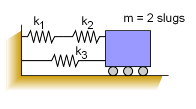Ch 10. Vibrations Multimedia Engineering Dynamics Free Vibs. Undamped Free Vibs. Damped Forced Vibration Energy Method
 Chapter - Particle - 1. General Motion 2. Force & Accel. 3. Energy 4. Momentum - Rigid Body - 5. General Motion 6. Force & Accel. 7. Energy 8. Momentum 9. 3-D Motion 10. Vibrations Appendix Basic Math Units Basic Equations Sections Search eBooks Dynamics Fluids Math Mechanics Statics Thermodynamics Author(s): Kurt Gramoll ©Kurt GramollDYNAMICS - EXAMPLESpring-Mass Motion Example What is the natural frequency of the system shown if k1 = k2 = k3 = 10 lb/ft? SolutionSpring Forces Stiffness constant of two springs in parallel,      F1 + F2 = F      x k1 + x k2 = x k12      k1 + k2 = k12 Stiffness constant of two springs in series,      x1 + x2 = x      F/k1 + F/k2 = F/k12      1/k1 + 1/k2 = 1/k12 For the current spring configuration, combine k1 and k2 (series) first,      1/k12 = 1/k1 + 1/k2              = 1/10 + 1/10      k12 = 5 The combined k12 and k3 (parallel),      k122 = k12 + k3             = 5 + 10 =15 The natural frequency of the combined system is,      ω = (k122/m)0.5 = (15/2)0.5 ω = 2.739 rad/s

Practice Homework and Test problems now available in the 'Eng Dynamics' mobile app
Includes over 400 problems with complete detailed solutions.
Available now at the Google Play Store and Apple App Store.